# 8. Portfolio Optimization (Part 1)

## 8.1 – A tale of 2 stocks

We have spent a great deal of time and efforts towards understanding risk associated with a portfolio. Our discussion has brought us to a very important stage – it’s time we discuss portfolio optimization. Perhaps, a good start to this discussion would be to understand what portfolio optimization is all about and why it makes sense to optimize a given portfolio.

Before we proceed, let me ask you a question – what do you think is the overall portfolio return, considering a portfolio consists investment in Infosys and Biocon (equally weighted). Assume the expected return of Infosys is 22% and Biocon is 15%.

I know it sounds like a typical MBA class question, but this is an important question and you should know how to answer this question at this stage J

Since the portfolio is equally weighted across two stocks, it implies we invest 50% in Infosys and 50% in Biocon. Given this, the expected portfolio return would be –

= Weight of investment in Infosys * Expected return of Infosys + Weight of investment in Biocon * Expected return of Biocon

Do recall, in the previous chapter we did discuss “Expected Return of a stock” in detail. Anyway, let us work out the answer –

50% * 22% + 50% * 15%

=11% + 7.5%

= 18.5%

So, the portfolio is expected to yield a return of 18.5% annually.

Great, now what if we change the weights? What if invest 30% in Infosys and 70% in Biocon? Or let us say 70% in Infosys and 30% in Biocon?

Let’s figure this out, Case 1 –

30% * 22% + 70% * 15%

= 6.6% + 10.5%

17.1%

Case 2 –

70% * 22% + 30% * 15%

=15.4% + 4.5%

=19.9%

Needless to say, we can do this for multiple combinations of weights. In fact, here is the table with few of the other combinations possible –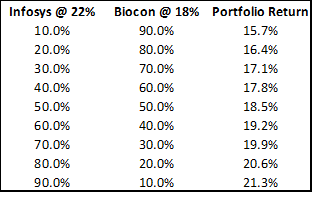As you can notice, as the investment weight varies, the returns also varies. For example if I had decided to invest just 40% in Infy and 60% in Biocon, I’d have enjoyed a return of 17.8%. However, if I had reversed it by investing 60% in Infy and 40% in Biocon, I’d have enjoyed a return of 19.2%, which is an additional 2% return.

This leads us to a super important conclusion – as the investment weights vary, the returns vary. In fact, each return has an associated risk profile, so it is prudent to state – as the weights vary, both the risk and return characteristics vary.

Now imagine this – for a given portfolio with ‘n’ number of stocks, wouldn’t it be awesome if you were to look at the past data and intelligently identify how much to invest in each stock, so that the portfolio yields the best possible returns?

This is exactly what happens when you optimize your portfolio. Generally speaking, you can adjust the weights (or optimize your portfolio) such that, for the given set of stocks –

• You identify the investment weights to achieve the best possible return or
• You identify the investment weights to achieve the least possible risk

## 8.2 – Caution! Jargons ahead

Hopefully by now, you fully appreciate the reason why one should optimize the portfolio. So, I won’t spend more time convincing you ☺

Let us go ahead and optimize the portfolio we have been working with. However, there are few important terms I want you to be familiar with at this stage –

Minimum variance portfolio – Assume you have a portfolio of 10 stocks. It must be quite obvious by now that you can play around with the weights of each stock to achieve different results. When I say results, I’m talking about the risk and return characteristics.  Each unique set of weights represents a unique portfolio. For example an equally weighted portfolio (10 stocks, 10% weight in each) is a unique portfolio. A portfolio where you invest 30% in stock 1 and 7.8% each across the remaining 9 stocks is another unique portfolio. The number of combination possible are many and each combination of weights results in a unique risk and return characteristics.

Given this, there should be that one set of combination of stock weights possible, such that the risk for the portfolio is the least possible. More technically, there should be combination of weights possible such that the variance of the portfolio is minimum. This particular portfolio is also referred to as the “Minimum Variance Portfolio”. The minimum variance portfolio represents the least amount of risk you can take. So if you are a highly risk averse investor, you should aim to create a minimum variance portfolio.

Maximum Return portfolio – This is somewhat the opposite of a minimum variance portfolio. Just like a minimum variance portfolio, there should be a combination of weights such that we can achieve a portfolio with maximum return possible. This also means that for a maximum return portfolio, the risk too will be on the higher side.

Fixed variance, multiple portfolios – This is not really a jargon, but a concept that you need to be aware of at this stage. It may come across as a little confusing at this point, but I’m certain, later on in this chapter (or maybe next) you will understand this much better, especially when we perform portfolio optimization.

For a given level of risk or variance of a portfolio, you can create at least two unique portfolios. One of such portfolio will yield the highest possible return and the other portfolio will yield the lowest return for the same given level of risk.

Here is an example on a completely arbitrary basis – let us say the risk or variance of a portfolio is 15%, given this, there will be a portfolio which can yield 30% return (highest possible return) and another portfolio which can yield 12% return (lowest possible return).  Do note, for both these portfolios, the risk is fixed to 15% but the returns vary.

Between these two portfolios there could be multiple other (unique) portfolios with varying return profile.   In super simple terms – for a fixed amount of risk, there could be multiple portfolio combinations, and within these possible combinations, there will be a portfolio with maximum return and another with minimum return.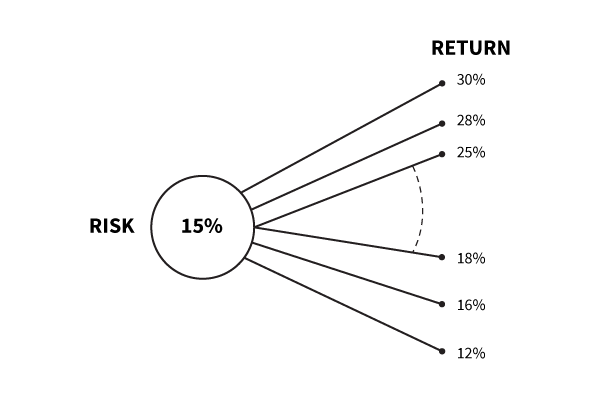We will revisit this concept a little later in the chapter, but for now, just keep this thought at the back of your mind.

## 8.3 – Portfolio optimization (steps)

Let us recall the portfolio that we have – the stock names and the associated weights are as follows. Do note, this is a continuation of the portfolio we have been working with over the previous few chapters.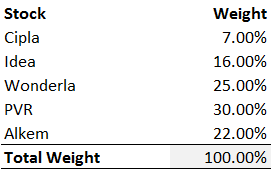Do remember, the weights assigned are all random, there was no thought process to it. For this portfolio with these combination of weights, the annual portfolio variance was estimated at 17.64% and expected return as 55.14%.

Our objective now is to optimize this portfolio to achieve a desired outcome. To optimize a portfolio in excel, we need the ‘Solver Tool’ in excel. You will find the solver tool under the ‘Data ribbon’.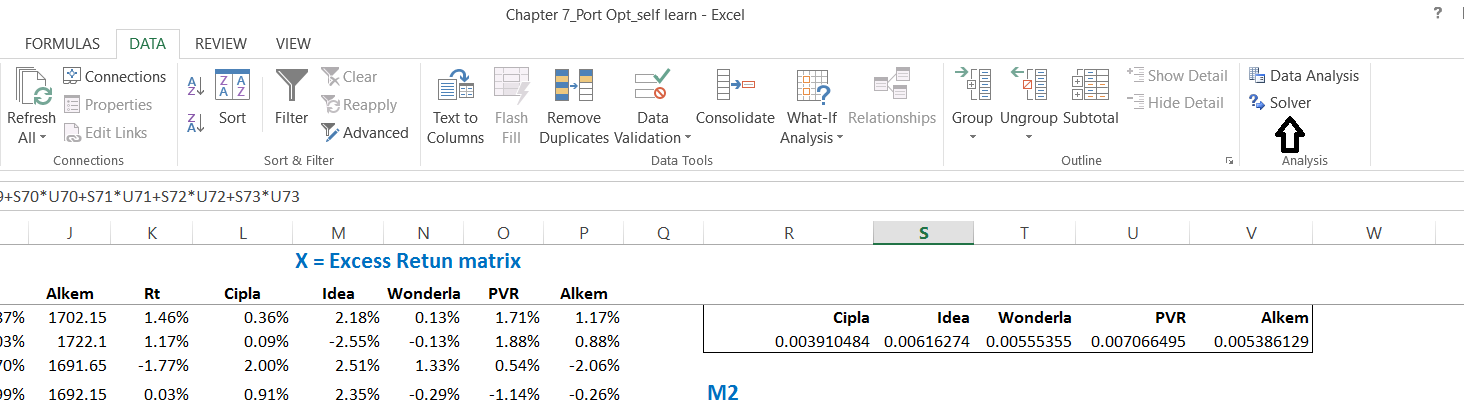Many of you may not find the ‘solver’ tool under the data ribbon. This is because you’ve not added it from the excel add ins. To add solver, follow these simple steps –

1. Click on Files on the opened excel sheet
2. Select Options
3. Select Add-ins (last but one option)
4. Click on ‘Solver Add Ins’
5. Click on “Go”
6. Check on “Solved Add ins” once again
7. Click Ok and close
8. Close the excel sheet, if required restart your system
9. Check under data ribbon – you should be able to find the solver tool

To begin with, let us optimize the portfolio to get the “Minimum Variance portfolio”. Here are few simple steps that you can follow to achieve this.

Step 1 – Organize your data. This is the key to using solver. Your cells should be linked, data should be neatly organized. No hard coding of data. Here is how the data on excel sheet looks at this stage.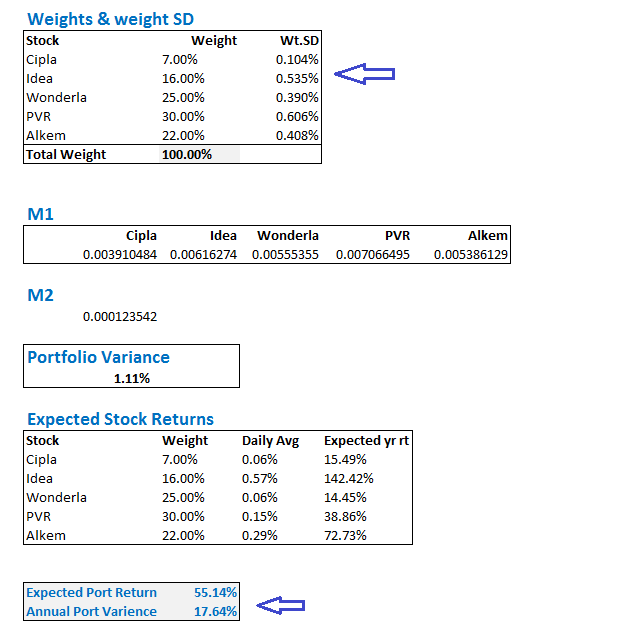I’ve highlight two important parts, which we will use for optimizing. The top most part has the weights assigned to each stock. Needless to say, this will change once the portfolio is optimized. The 2nd part has the expected return and annual portfolio variance calculation, which will also change when we optimize the portfolio.

Step 2 – Use the solver tool in excel to optimize the weights. I’m assuming you may be new to solver, hence will give you a quick overview of this tool. You can use solver to work with something called as an ‘objective’. An objective, according to solver is essentially a data point, derived by set of formulas. You can minimize the objective’s value or maximize the objective’s value or set the value of an objective to a certain desired value. You can do this while changing certain variables. The variables, according to solver are the elements of the formulas used in deriving the objective.  For example, I can choose to minimize the variance of the portfolio by changing the weights of each stock. Here, the variance is the objective and the weights are the variable.

When we command the solver to minimize the objective (variance in this case), then in the background, excel’s solver will quickly check the formulas used and works around it in such a way that the objective’s value is least minimum.

Look at the image below, I’m invoking the solver tool and will soon ask it to minimize the variance.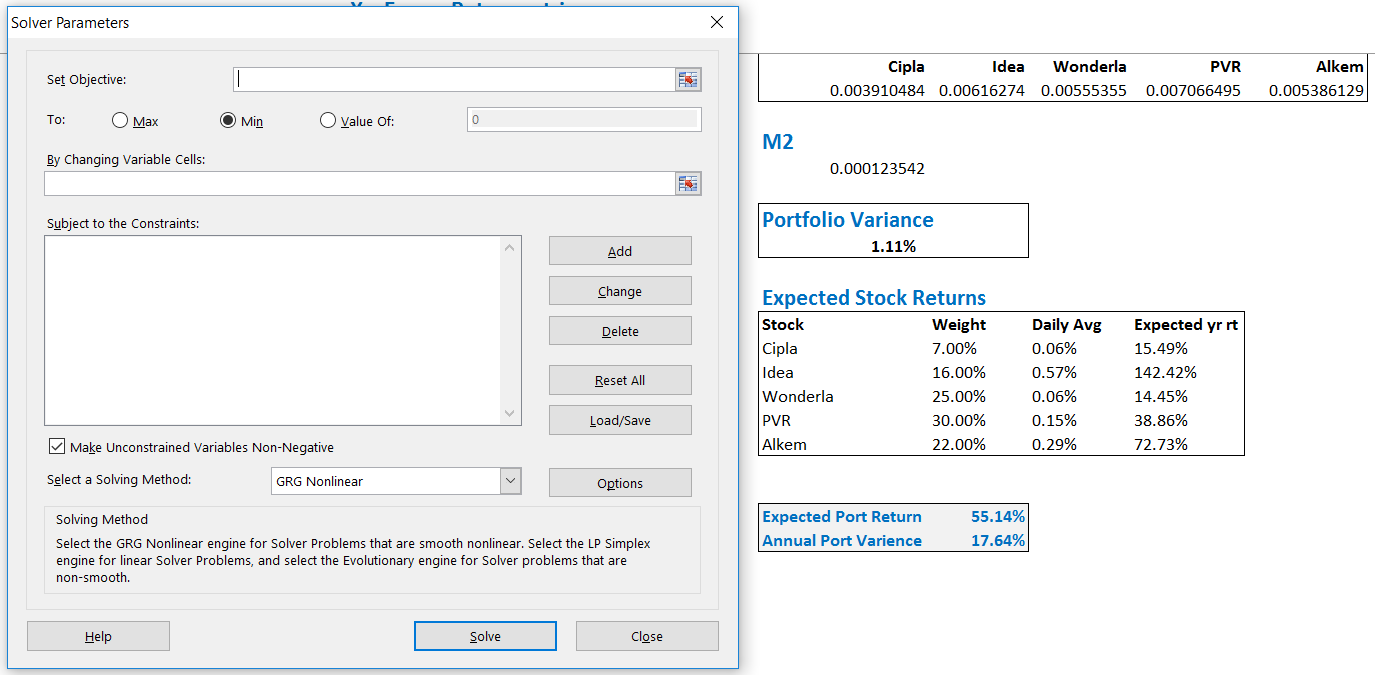When you click on the data ribbon and click on solver, you will see the solver tool open up, as seen above. We need to set the objective here. Objective as I mentioned earlier, is the annual portfolio variance. Remember, we are working towards finding the minimum variance portfolio here.

Check the image below –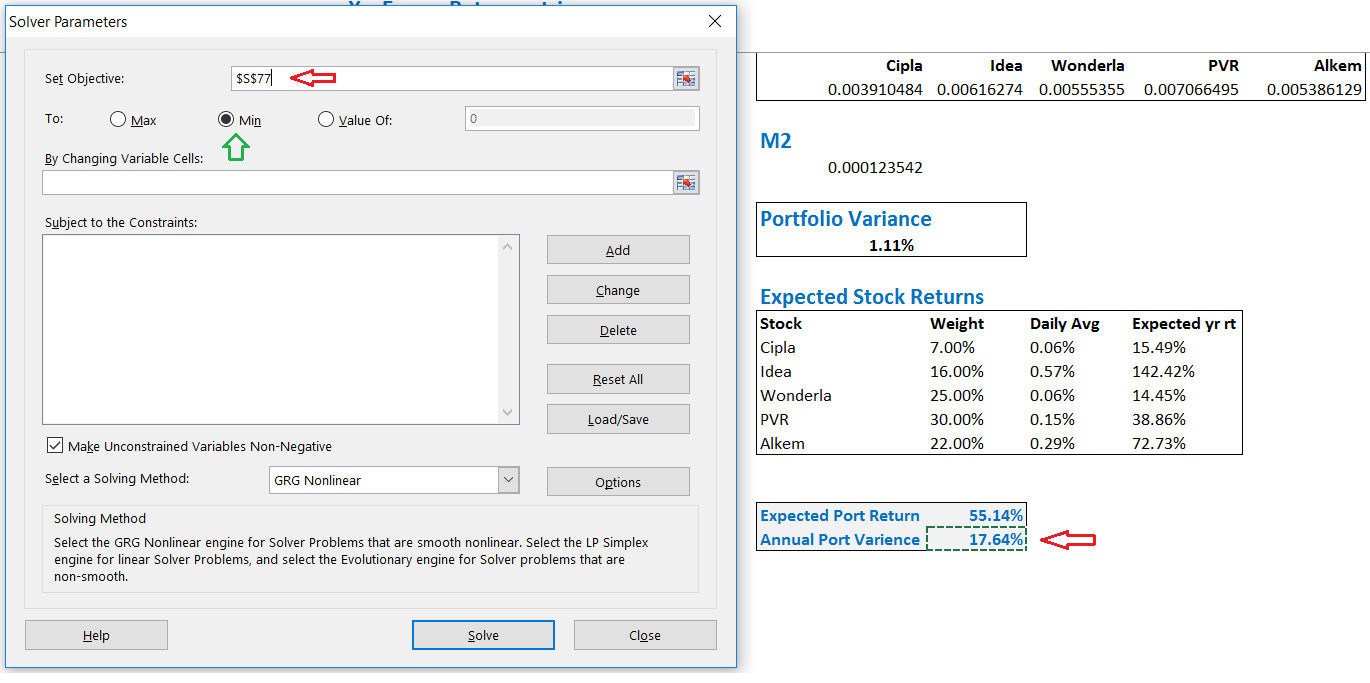Objective is set to ‘Annual portfolio variance’ – you can notice the cell address is highlighted in the ‘set objective’ field. The cell containing the annual portfolio variance itself is highlighted below, you will find another red arrow here. We are minimizing the objective here, the same is highlighted by the green arrow.

Once this is set, the next step is to inform the solver tool that we need minimize the objective by changing the variables. In this case, the variable happens to be the weights assigned to each stock.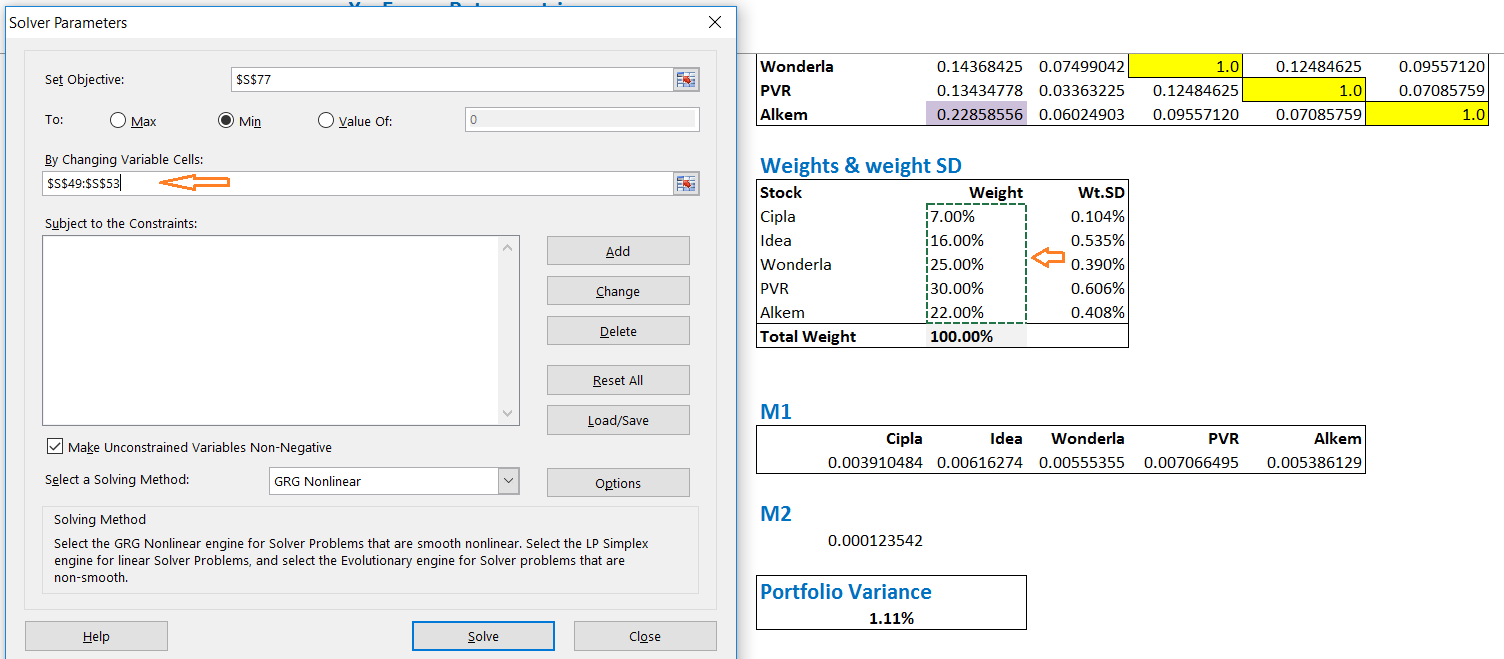As you can see, in the “By changing variable cells” field, I’ve highlighted the weights assigned to each stocks.

You can also find “subject to constraints”, field. This essentially means, that solver will minimize the variance, by change the weights of each stock, and at this stage, it is also asking us if there are any constraints it needs to keep in mind while solving to minimize the variance.

One constraint that I can think of at this stage is that the total weight of all stocks put together should be 100%. This essentially means that my capital is 100% deployed across all the 5 stocks. If I do not specific this, then there is a chance that solver may suggest to skip investments across few stock altogether. Remember, solver is an excel tool, and it does not appreciate stock picking

To add a constraint, click on ‘add’. When you do so the following window opens up –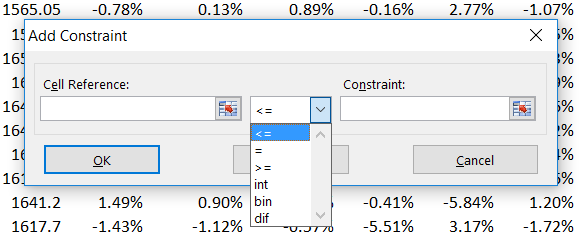Under Cell reference, I will give the sum of weights of stocks – which needs to be equal to 100%. Next to this, you can see a drop down menu with multiple options, I’d pick ‘=’ here. Finally, the constraint itself will be 100%. Note, I’ve typed out 100% here.

In simple words, I’m asking solver to optimize for minimum variance, keeping the weight of all stock to 100%. The window now looks like this –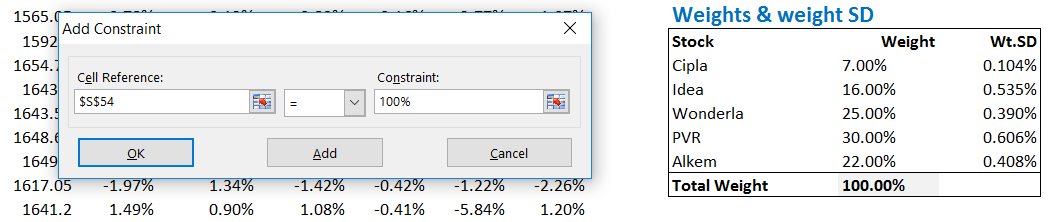The solver is completely set up now. The final screen before pressing “Solve” looks like this –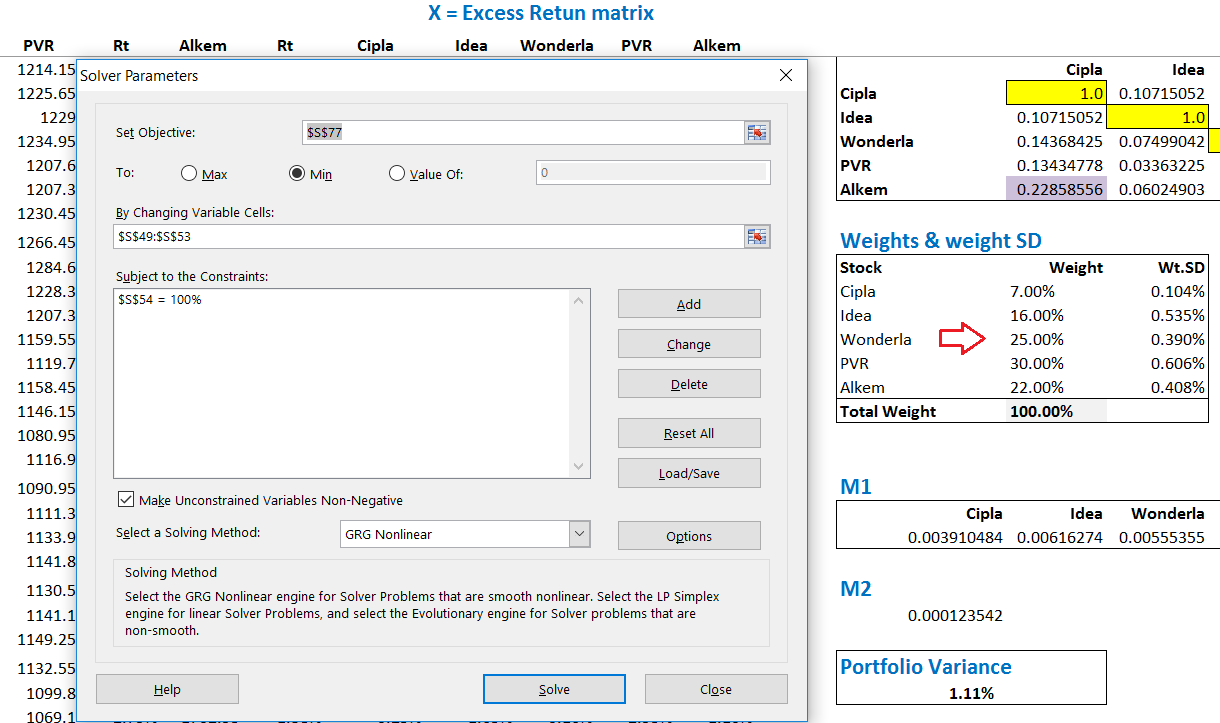I’ve highlighted the weights of each stock for reference. Remember, these are pre optimized weights that we randomly assigned at the beginning of this discussion. Post optimizing, the weights will be changed such that the variance is least possible for these set of stocks. Let us go ahead and press ‘solve’ and check what solver has for us.

And here you go –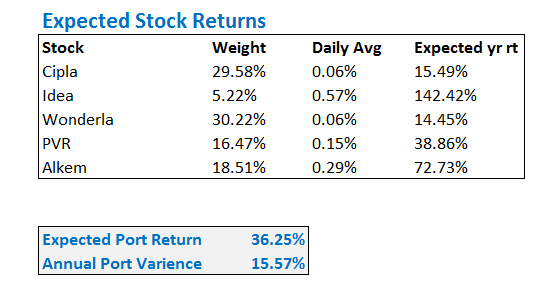Solver has solved for the ‘minimum variance’ portfolio and accordingly it has worked out the weights for each stock.

For example, it wants us to increase the weight in Cipla from current 7% to 29.58%, while it wants us to reduce the weight in Idea to 5.22% from 16%. So on and so forth. Further, it is also telling us that the least possible variance with this portfolio is 15.57% (remember, the variance was earlier at 17.64%).  Along with this, the portfolio’s expected return too seem to have dropped to 36.25% from the earlier 55.14%.

So, no matter what you do, the variance cannot be lowered below 15.57%. In other words, if these are the 5 stocks that you want to invest in, then the least amount of risk you will be exposed to is 15.57% and absolutely nothing below that!

I’ll leave you at this. In the next chapter, we will optimize the same portfolio for few more scenarios and work towards building something called as an ‘Efficient Frontier’.

You can download the excel sheet used in this chapter. Do note, the excel contains the optimized weights for the minimum variance portfolio.

### Key takeaways from this chapter

1. The returns of the portfolio is dependent on the weights assigned to each stock
2. Minimum variance portfolio is that portfolio where the variance or risk is least possible for the given set of stocks
3. Maximum return portfolio is that portfolio where the expected portfolio returns are maximized for the given set of stocks
4. When we fix the variance of a portfolio we can achieve at least two portfolios where the expected portfolio returns can be maximum or least
5. One can optimize a given portfolio with ‘n’ number of stocks on excel, by using the solver tool
6. One of the most important points to remember while using solver is to ensure the data is well organized. One can do this by linking all the relevant cells and avoiding hard coding of values
7. You can optimize the portfolio by subjecting the variable to constrains

1.Santosh Shetti says:

Dear Karthik,
Wow, that’s Awesome! Simply Awesome!

Have a question, In our example, we used solver for Minimum Variance and the result was approx 2% reduction in exposure to risk, but at the same time Expected return has also reduced drastically from 55.14% to 36.25%.
So on excel sheet provided by you, I tried something i.e. I added one more constraint where I restricted Expected Returns equal to our original 55.14% and as a result annual Portfolio variance came to 16.9% which is greater than 15.57% but still lower than original 17.64%.

Is this approach, in a right direction? Waiting for your valuable feedback.

Thanks & Regards
Santosh Shetti 🙂

•Karthik Rangappa says:

Of course! Optimization is all about this. Remember, you can either work with the variance or returns, given this your approach is perfectly valid.

•Santosh Shetti says:

Thanks a lot.

I have one more question, which pertains to historical data. Like if I decided to take past one year closing price into consideration while calculating port portfolio variance, but what if couple of stocks in portfolio doesn’t have adequate historical data (as those stocks have been recently listed), can we go with whatever little data is available or we do need to make some adjustments?

Thanks & regards ☺

•Karthik Rangappa says:

That would be a problem :).

For example, if I have a recently listed stock in my portfolio, say 6 months, then I’d take the historical data for 6 month for all the other stocks as well. Alternatively, I wont hesitate to keep this one stock aside and optimize the rest of the portfolio.

•Santosh Shetti says:

Ok got it. Thanks a lot Karthik. 🙂

•Karthik Rangappa says:

Cheers!

2.Varun says:

Thanks for all the tutorials Karthik Sir.

I wanted a overview on what topics/content are you going to cover in Trading strategies and systems.

•Karthik Rangappa says:

We are yet to finalize them, Varun. We are currently focused on delivering a good module on Risk Management and trading psychology.

3.VIVEK KUMAR SINGH says:

thanks for this great tutorials

•Karthik Rangappa says:

Cheers!

4.zb2134 says:

Hi karthik,
from where should I collect the data of stocks closing price for last 6 months to find out variance of my portfolio. Is it available on zerodha platform? Really you people are doing great job by educating people and responding promptly keep it up.

•Karthik Rangappa says:

•zb2134 says:

Hi Karthik,
I am finding it quiet cumbersome to calculate my portfolio variance and risk profile using your sample excel sheet and downloading data from NSE website. Does zerodha provides the facility for the same to its customer for the portfolio holding?

•Karthik Rangappa says:

Not as of now. The trick is to make sure that the data is neatly organized on excel.

5.ZV9780 says:

hi Karthik,
we are not able to see module 7 and 8 in the dropdown.

•Karthik Rangappa says:

I’m able to see, can you please do a hard refresh and check again? Thanks.